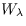Next: 8.2.3 1995 Wavelength Calibration Up: 8.2 Low-Dispersion Wavelength Calibration Previous: 8.2.1 Parameterization of the

8.2.2 Application of the Dispersion Relations

The dispersion solutions derived from individual WAVECAL images display variations from the averaged dispersion solutions discussed above. These variations arise from two sources, the first of which is simply the measurement uncertainties involved in locating the Pt-Ne emission features in the WAVECAL images and the corresponding uncertainties in the coefficients of the derived dispersion solutions. Second, global shifts in the location of the spectral format within individual images are known to occur as a function of camera temperature (THDA) and time (see e.g., Thompson 1988 and Garhart 1993). These shifts occur both parallel and perpendicular to the dispersion direction and consequently result in changes to the wavelength zeropoint (A1) as well as the spatial location (B1) of spectra within the low-dispersion SI. These format shifts appear to be a translational shift only, with no change in image scale. As a result, the higher order dispersion term (A2) remains constant.

The dispersion coefficients as actually applied to science images are determined by adding to the mean zeropoint terms corrections appropriate for the observation date and THDA of the particular image. The correction termsand Ws, which represent the offset that is added to the mean wavelength and spatial zeropoints, are defined by the following general expressions:Ws = W1s + W2st + W3sT + W4st2 + W5st3

whereand Ws are the corrections to be added to the A1 and B1 terms of the mean dispersion relation, respectively, T is the THDA at the end of exposure, and t is time expressed as the total number of elapsed days since 1 January 1978.

A first-order (i.e., linear) fit is sufficient to characterize the correlation between THDA and zeropoint for all cameras. The correlation between time and zeropoint, however, has second- and third-order dependencies for some cameras. Theand Ws coefficients in use for the low-dispersion mode for pre-1990 LWP and SWP images are listed in Table 8.2. Coefficients appropriate for more recent LWP and SWP images are discussed in Chapter 8.2.3. The database of exposures used to generate the coefficients in Table 8.2 include WAVECAL images obtained through mid-1993 for the LWP and mid-1991 for the SWP. The respective coefficients for the LWR camera are listed in Table 8.3. In the case of the LWR, a separate set of coefficients are tabulated for the ITF A and ITF B calibrations. In addition, each ITF has an early and late epoch. This is due to the fact that the wavelength zeropoint shift was dramatically different between these two epochs. The cutoff date for the early and late epochs is 1980.1 for ITF A and 1979.9 for ITF B. The shift of the spectrum center does not exhibit this trend, so the spatial coefficients are identical for each epoch. The zeropoint shifts and corresponding polynomial fits are illustrated graphically for each camera in Figures 8.2-8.5.Next: 8.2.3 1995 Wavelength Calibration Up: 8.2 Low-Dispersion Wavelength Calibration Previous: 8.2.1 Parameterization of the
Karen Levay
12/4/1997